### Day 20 - Product/Quotient Rule - 02.01.16

 Update Unit 2 TestFriday, 02.12.16Calculus Equations/TheoremsDerivative Operator Find the derivative of the following: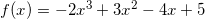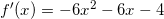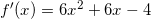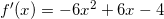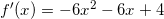none of the aboveFind the second derivative (derivative of the derivative) of the following: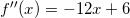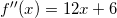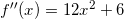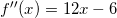none of the aboveFind the point(s) at which the following function has a horizontal tangent line: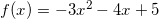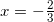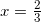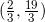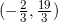none of the aboveFind the derivative of the following function using the Product Rule: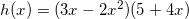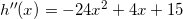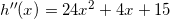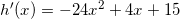none of the aboveFind the derivative of the following function: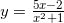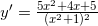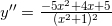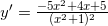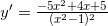none of the aboveReviewPre-calculusSlopeEquation of a LineSecant Line vs. Tangent Line (video)DerivativeHow can the slope of a tangent line be found? Equation of a Tangent Line (video)Tangent Line GraphDerivative (video)CheckpointsBasic Differentiation RulesConstant Rule (video) (example)Power Rule (video) (example)Constant Multiple Rule (video) (example)Sum and Difference Rule (video)Derivative of Sine and Cosine FunctionsCheckpointsLessonProduct Rule (video) and Quotient Rule (video)How can derivatives of the product/quotient of functions be calculated?Checkpoints Exit Ticket Posted on the board at the end of the block.HomeworkWNQ the following:Chain Rule (video)Study! Help Request List Standard(s) APC.2Define and apply the properties of limits of functions.Limits will be evaluated graphically and algebraically.Includes:​limits of a constant​limits of a sum, product, and quotient​one-sided limits​limits at infinity, infinite limits, and non-existent limitsAPC.3Use limits to define continuity and determine where a function is continuous or discontinuous.Includes:​continuity in terms of limitscontinuity at a point and over a closed interval​application of the Intermediate Value Theorem and the Extreme Value Theorem​geometric understanding and interpretation of continuity and discontinuity